/

### Elliptical distributions

An elliptical distribution is a multivariate distribution whose (multivariate) characteristic function,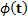,being a vector with the same number of entries as there are dimensions for the distribution, of the form: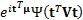where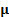is a specified variable, and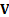is a positive definite matrix.

If the distribution has a probability density function then it will take the form: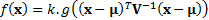where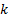is a scale factor,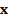is an n-dimensional random vector with median vector(which is also the mean vector, if the latter exists), is a positive definite matrix which is proportional to the covariance matrix if the latter exists, and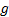is a function mapping non-negative real numbers to non-negative real numbers with finite area under the curve.

Perhaps the best known elliptical distributions are multivariate normal (i.e. Gaussian) distributions.